# The Azimuth Project Blog - connections: Petri nets and beyond (part 1)

This page is a blog article in progress, written by Ken Webb. To see discussions of this article while it is being written, go to the Azimuth Forum.

guest post by Ken Webb

I like knowing how things are connected. I hate when I find a new concept, sense that concept is connected to something I already know, but have no idea how to confirm my intuition. The people and writings at the Azimuth Project have introduced me to multiple new things. In this blog I explore one of these, Petri nets, and how they can help build connections to some of the islands in my knowledge.

Unlike many people here, I’m not good at math. Instead I depend on my software development skills. My approach in this blog is to build a simple executable Petri net with software, and then convert it to other formats that can then be processed using tools from other domains. The Petri net example can be viewed as a very non-mathematical mind map, processed by (at least) several Petri net tools, executed by various tools as a system of differential equations, displayed as a Unified Modeling Language (UML) class diagram and as a UML sequence diagram, converted into System Biology Markup Language (SBML) so it can be processed by numerous other tools from the biology community, and run in a rectangular grid as an agent based model (ABM). But this initial article restricts itself to building a bridge from Petri nets to differential equations.

Another motivating factor is that I’ve looked for simple climate models for a long time but haven’t found anything satisfactory either online or in books or periodicals. Many use some flavor of differential equations, and I don’t know how to build these from scratch and/or have no access to the source code. Much of the information available online seems contradictory or incomplete when you actually get down to trying to put it into a programming language. By simple, I mean something that a generally well-educated person can understand, can interact with, and can even help to build. I’ve made various attempts to build my own models (see examples here toward the end of the page and here ) based on what I’ve found, but I’ve been lacking a solid base to start from. Although Petri nets aren’t enough, they do appear to provide the base I’ve been looking for. The rest of this article is about firming up the base in preparation for future climate modeling.

I want to start with a qualitative model that can readily be transformed into something more and more quantitative. I want to think about the things in the model before having to consider the actual numbers of things. For example, the Simple Climate Model in this set of Petri net experiments is strictly qualitative. All arc weights are 1, so all it does is demonstrate the sorts of things that get moved around. And yet it does convey some of the basic concepts, and it can be extended to be more quantitatively accurate.

### Petri nets and reaction networks

Petri nets have been well described elsewhere at the Azimuth Project, such as here, here, and here. Basically, a Petri net consists of a set of nodes and a set of edges that connect the nodes. The nodes are of two types, place nodes (sometimes called states) and transition nodes. An edge is called an arc. Each place node contains zero or more tokens. Places only connect to transitions, and transitions only connect to places. Transitions act on the tokens in the places they connect to, by moving tokens from input places to output places.

In the following Petri net diagram, the filled rectangles are transitions, the circles are places, and the numbers inside the circles are token quantities. Each transition and place has a unique label. Each arc has an arrow head that shows if it’s an input arc (from a place to a transition) or an output arc (from a transition to a place). The arcs are labeled with numbers called weights (1 or 2 in the diagram).

Xholon (a tool we’ll get to soon) wrote this out as a Petri Net Markup Language (PNML) file. The PIPE2 Petri net tool displays it as the following, after some manual moving around of the nodes.

Diagram created by PIPE2 from Xholon data.Reaction networks were independently developed, but are almost exactly the same as Petri nets. These are defined and described in Part 21 of John Baez’ network theory course, where they are given a more mathematically precise treatment. Part 21 uses the same Feinberg reaction network that we’re using as an example in this article. A reaction network uses the terminology species and reaction rather than place and transition. A reaction involves two or more of the arcs in the Petri net. An excellent early source of information on reaction networks are Martin Feinberg’s lectures where they are called chemical reaction networks (CRN). The following diagram shows Feinberg’s first example reaction network, which is the same as the Petri net above. The reaction labelled ξ is the same as transition BE_AC in the Petri net. It converts one unit of B and one unit of E into one unit of A and one unit of C. The combinations of species B+E and A+C are called complexes. In this example, B+E is a source complex, and A+C is a target complex.

Xholon is a general-purpose Java-based modeling and simulation tool I’ve written. After being inspired by glowing accounts of Petri nets and reaction networks at the Azimuth Project, I added a Petri net and reaction network plug-in to Xholon. Xholon models are typically specified as XML hierarchical structures, with additional links between nodes. Xholon is pronounced the same as holon, a very relevant concept from philosophy.

The following is an example of a Petri net specified in Xholon XML format. It’s an implementation of the Feinberg Petri net and reaction network described above. There are five places (A B C D E) each with a specified initial number of tokens. There are six transitions (A_BB BB_A AC_D D_AC D_BE BE_AC), each with a displayable symbol (the Greek letters in the reaction network). Each transition has one or more input arcs and output arcs. Each arc has a weight and connects to one place node. The connectors identify the place using an XPath expression, which provides directions on how to get from the InputArc node to the place node. For example, ancestor::PetriNet/Places/A means locate an ancestor node called PetriNet, locate a child of that node called Places, and then a child of that node called A.

<?xml version="1.0" encoding="UTF-8"?>
<ReactionNetworkSystem>
<PetriNet roleName="Feinberg 1.1" kineticsType="1" p="1.0">
<QueueTransitions/>
<AnalysisPetriNet/>
<AnalysisCRN/>
<AnalysisCat/>
<Places>
<A token="140"/>
<B token="180"/>
<C token="200"/>
<D token="25"/>
<E token="80"/>
<Plot mode="new"/>
</Places>
<Transitions>
<A_BB k="0.0039" symbol="α">
<InputArcs>
<InputArc weight="1" connector="ancestor::PetriNet/Places/A"/>
</InputArcs>
<OutputArcs>
<OutputArc weight="2" connector="ancestor::PetriNet/Places/B"/>
</OutputArcs>
</A_BB>
<BB_A k="0.0038" symbol="β">
<InputArcs>
<InputArc weight="2" connector="ancestor::PetriNet/Places/B"/>
</InputArcs>
<OutputArcs>
<OutputArc weight="1" connector="ancestor::PetriNet/Places/A"/>
</OutputArcs>
</BB_A>
<AC_D k="0.0037" symbol="γ">
<InputArcs>
<InputArc weight="1" connector="ancestor::PetriNet/Places/A"/>
<InputArc weight="1" connector="ancestor::PetriNet/Places/C"/>
</InputArcs>
<OutputArcs>
<OutputArc weight="1" connector="ancestor::PetriNet/Places/D"/>
</OutputArcs>
</AC_D>
<D_AC k="0.0036" symbol="δ">
<InputArcs>
<InputArc weight="1" connector="ancestor::PetriNet/Places/D"/>
</InputArcs>
<OutputArcs>
<OutputArc weight="1" connector="ancestor::PetriNet/Places/A"/>
<OutputArc weight="1" connector="ancestor::PetriNet/Places/C"/>
</OutputArcs>
</D_AC>
<D_BE k="0.0035" symbol="ε">
<InputArcs>
<InputArc weight="1" connector="ancestor::PetriNet/Places/D"/>
</InputArcs>
<OutputArcs>
<OutputArc weight="1" connector="ancestor::PetriNet/Places/B"/>
<OutputArc weight="1" connector="ancestor::PetriNet/Places/E"/>
</OutputArcs>
</D_BE>
<BE_AC k="0.0034" symbol="ξ">
<InputArcs>
<InputArc weight="1" connector="ancestor::PetriNet/Places/B"/>
<InputArc weight="1" connector="ancestor::PetriNet/Places/E"/>
</InputArcs>
<OutputArcs>
<OutputArc weight="1" connector="ancestor::PetriNet/Places/A"/>
<OutputArc weight="1" connector="ancestor::PetriNet/Places/C"/>
</OutputArcs>
</BE_AC>
</Transitions>
</PetriNet>
</ReactionNetworkSystem>

At this point we have three descriptions of the same thing - a Petri net diagram, a reaction network diagram, and an XML structure.

### Kinetics

Note the third line down in the XML:

<PetriNet roleName="Feinberg 1.1" kineticsType="1" p="1.0">

The XML attribute kineticsType=“1” means that the Petri net obeys the traditional rules of a place/transition net. It has kinetics type 1. Each time step, each transtion checks its input arcs. If all input arcs have a weight that’s greater than 0.0, then the transition fires. When it fires, it decreases the number of tokens in each input place by the amount specified in the corresponding input arc, and it increases the number of tokens in each output place by the amount specified in the corresponding output arc. For example, transition BB_A will decrease the number of tokens in place B by 2, and will increase the number in A by 1 token.

The XML attribute p=“1.0” means that the Petri net does the transitions with 100% probability. If p=0.0 then nothing will happen. If p=0.5 then the transitions will fire an average of 50% of the time steps. The same probability p can be set for all transitions as is done here, or each transition can have its own p value.

Xholon also randomizes the firing order of transitions each time step. So even if all transitions fire each time step, AC_D may fire first in time step 23 while AA_B may fire first in time step 24.

With kineticsType=1 and p=1.0, the Xholon simulation of the Petri net generates a line graph that looks similar to the following.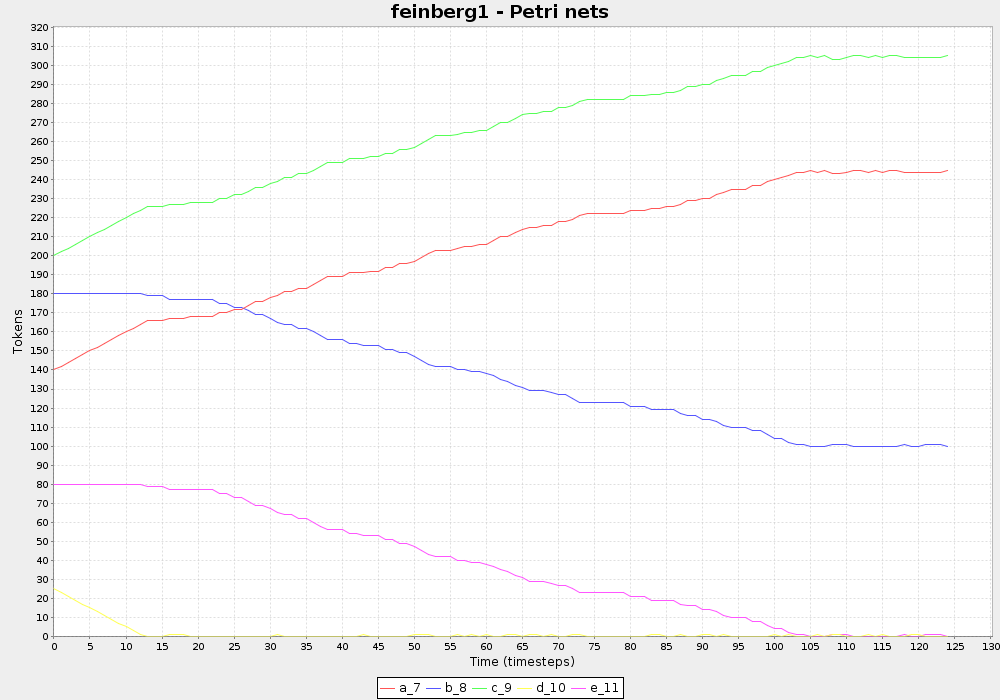With kineticsType=1 and p=0.1666666667, the line graph shows a lot more variabililty. With this p value, there is an average 1 in 6 probability that each of the 6 transitions will fire each time step. So on average only one transition will fire each time step.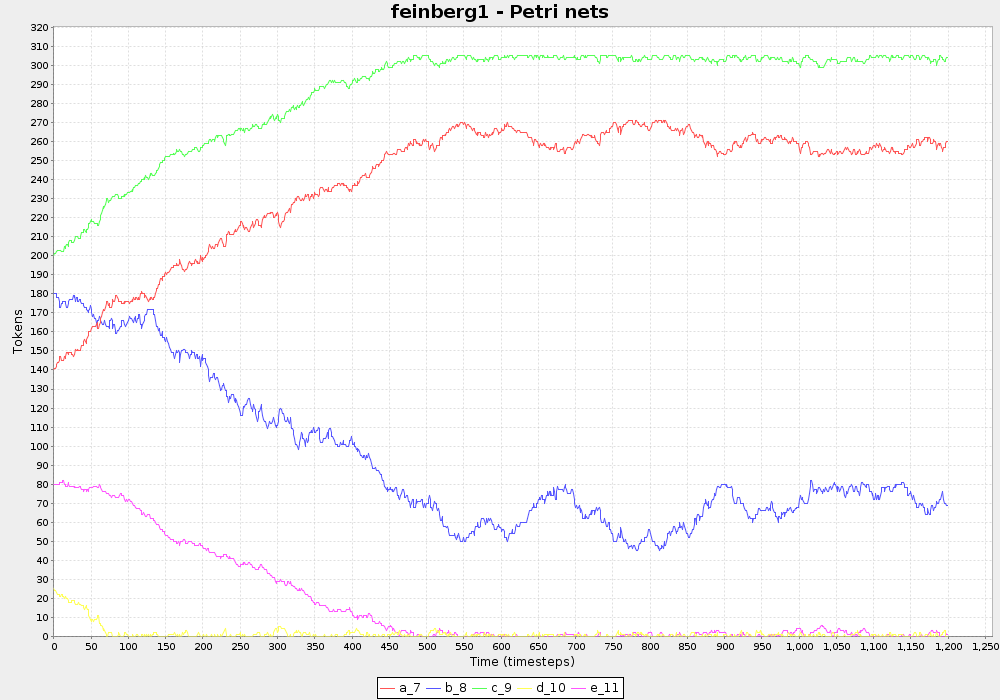Xholon can transform the model into Python syntax, so it can be executed by David Tanzer’s Petri net software.

# To run this Petri net (in Ubuntu Linux):
# (1) Save this python script to a .py file, for example pn_test.py .
# (2) Ensure that David Tanzer's petri1.py is in the same directory as this script.
# (3) Open a terminal window.
# (4) Run:
#     python pn_test.py
# (5) Or run it while redirecting the output to a CSV file:
#     python pn_test.py > pn_test.csv

from petri1 import *

# Petri net - Feinberg Lectures 1.1
A_BB = ("A_BB", [["A"]], [["B",2.0]])
BB_A = ("BB_A", [["B",2.0]], [["A"]])
AC_D = ("AC_D", [["A"], ["C"]], [["D"]])
D_AC = ("D_AC", [["D"]], [["A"], ["C"]])
D_BE = ("D_BE", [["D"]], [["B"], ["E"]])
BE_AC = ("BE_AC", [["B"], ["E"]], [["A"], ["C"]])
petriNet = PetriNet(
["A", "B", "C", "D", "E"], # states
[A_BB, BB_A, AC_D, D_AC, D_BE, BE_AC] # transitions
)
initialLabelling = {"A":140.0, "B":180.0, "C":200.0, "D":25.0, "E":80.0}
steps = 1200
petriNet.RunSimulation(steps, initialLabelling)

Running the Python version of the Petri net, and using a different graphing tool, produces a line graph similar to the following. It looks broadly similar to the Xholon line graph with p=0.1666666667 .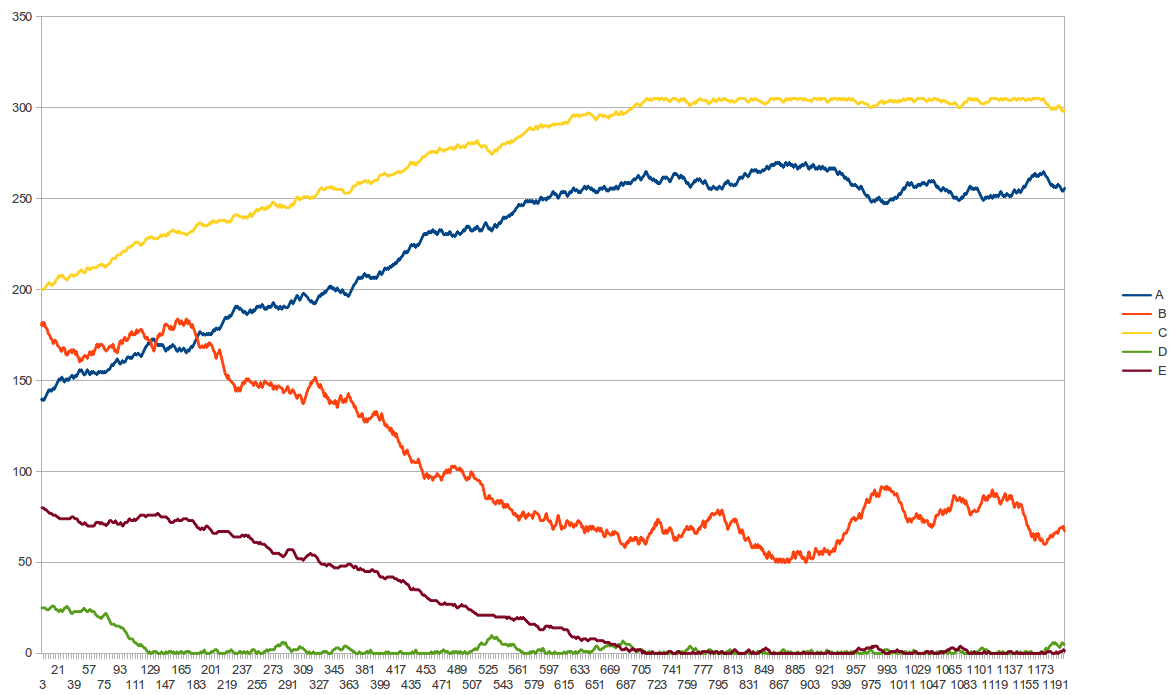A second type of kinetics is called Mass Action, and it’s typically used with reaction networks. If kineticsType=“2” in the XML model, then the Xholon simulation uses mass action kinetics rather than the traditional Petri net place/transition rules. Note that each transition in the XML has a k attribute, for example:

<A_BB k="0.0039" symbol="α">

The k value is a rate constant that helps determine the rate at which the transition transforms its inputs into its outputs each time step. For example, transition A_BB runs with a rate constant of 0.0039. Also, each transition fires at a rate that is proportional to the product of its input concentrations. So, to determine the current rate, we multiply the rate constant by the product of the current amounts in all of its input places. We then remove that number of tokens from each of the input places, and add that number of tokens to each of the output places. When a Petri net or reaction network uses rate constants with the transitions, then we refer to it as a stochastic Petri net or stochastic reaction network. Simulating this model using mass action kinetics, produces a line graph that should be identical to the following.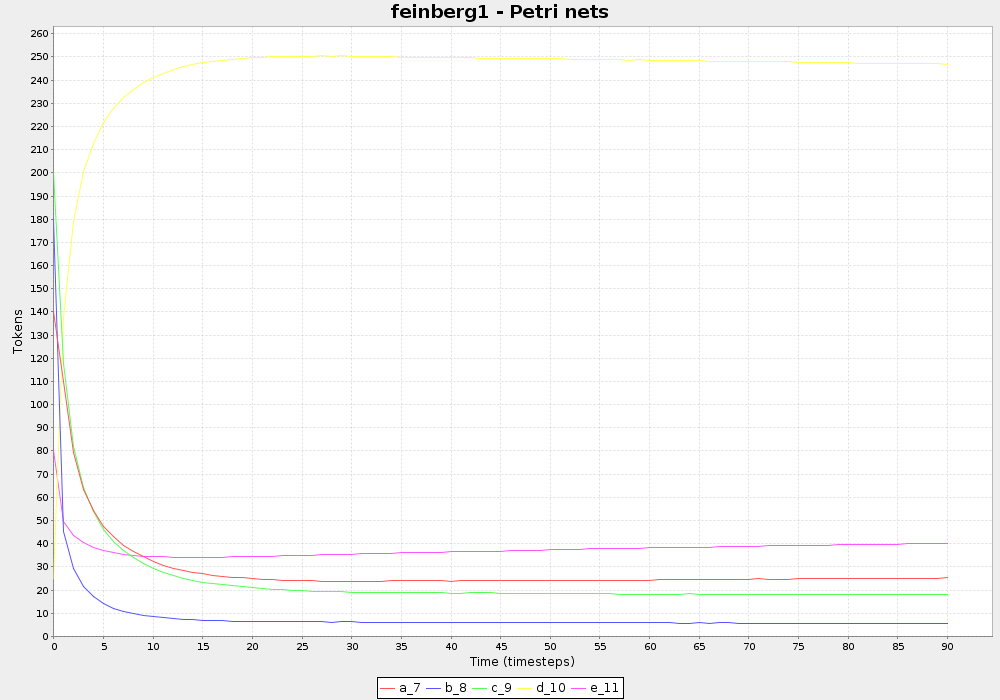Why use a k value of 0.0039? Because this is a made-up system, there’s no way to determine experimentally what it’s value should be. But if I use higher values, then the lines in the graph start to oscillate more and more, or go shooting off to positive or negative infinity. There’s no room to explain why that would happen, but perhaps in another blog. It has to do with calculus and finding the right small interval of time to use as a time step.

### From Petri nets to differential equations

Petri nets turn out to be a great starting point for modeling with other formalisms, including some I don’t understand very well. For example, a stochastic Petri net (a Petri net where the transtions have rate constants) can be automatically transformed into a system of differential equations. Let’s work through exactly what happens when we do this. If you don’t know or remember what differential equations are, then think of the following steps as a recipe, or driving instructions (how to get from Petrnet Town to Diffeq City), or maybe as a mathemagical incantation. This is something you can do by hand.

#### Step 1

Write the name of each place (A B C D E in this model) on a separate line. Then write d/dt  in front of each name, and  =  after it. d/dt  means rate of change, so d/dt A is the rate of change of the number of tokens in place A.

d/dt A =
d/dt B =
d/dt C =
d/dt D =
d/dt E = 

#### Step 2

For each place, look through all the transitions. When you find an input arc that involves that place, write  - at the end of the line. Write  + for an output arc. For example, A occurs five times in the six transtions, twice as an input and three times as an output. We say that A has five terms.

d/dt A = - + - + +
d/dt B = + - + -
d/dt C = - + +
d/dt D = + - -
d/dt E = + -

#### Step 3

Each transition has a rate constant (symbolized as the Greek letters α β γ δ ε ξ in this model). For each term, write the rate constant directly after the - or + symbol. Each rate constant may also be given a numeric value (0.0039 0.0038 0.0037 0.0036 0.0035 0.0034 in this model), but in this process we will use the symbol. If you hate having to write Greek symbols, then write alpha beta gamma delta epsilon xi instead, for example d/dt E = +epsilon -xi.

d/dt A = -α +β -γ +δ +ξ
d/dt B = +α -β +ε -ξ
d/dt C = -γ +δ +ξ
d/dt D = +γ -δ -ε
d/dt E = +ε -ξ

#### Step 4

Each transition has zero or more input arcs, where each arc references a place. For each term, write the names of all the places referenced by input arcs for that transition. If there is just one input arc, write the name of its place after the rate constant symbol. If there are two or more input arcs, write the names of all the places. These will all be multiplied with each other, so it’s OK to just write the single-character names of each place with no intervening spaces.

d/dt A = -αA +βBB -γAC +δD +ξBE
d/dt B = +αA -βBB +εD -ξBE
d/dt C = -γAC +δD +ξBE
d/dt D = +γAC -δD -εD
d/dt E = +εD -ξBE

#### Step 5

If an input arc has a weight greater than 1, for example 2, then you can write it in one of two ways. For example, BB or B^2, because B times B is the same as B to the power of 2 (B squared). The symbol ^ is often used to mean to the power of.

d/dt A = -αA +βB^2 -γAC +δD +ξBE
d/dt B = +αA -βB^2 +εD -ξBE
d/dt C = -γAC +δD +ξBE
d/dt D = +γAC -δD -εD
d/dt E = +εD -ξBE

#### Step 6

This is probably the step that’s the least intuitively obvious. I have problems with it. Any term that involves a transition where either the input arcs or output arcs have a weight greater than 1, may need a final adjustment. For example, if we’re figuring out the rate of change of B, then any term involving a transition that involves multiple instances of B, will need an adjustment. There are two such terms. Once I really get this, I’ll try to write it up in a more meaningful way. For now, the differential equation just works (I think) if we do things this way.

d/dt A = -αA +βB^2 -γAC +δD +ξBE
d/dt B = +2αA -2βB^2 +εD -ξBE
d/dt C = -γAC +δD +ξBE
d/dt D = +γAC -δD -εD
d/dt E = +εD -ξBE

So. This is our system of differential equations. The process we’ve just worked through has been captured as an algorithm in Java, a popular programming language. To see the algorithm, look at the getDifferentialEquations() and getTerm() methods in the AnalysisCRN Java class. Warning: If you’re not a programmer, then this may be even scarier than thinking about differential equations for those of us not very math inclined.

What good are differential equations? A simple thing we can do is to format them so they look the way that mathematicians and others expect to see them when displayed on a web page (or in a book). Xholon automatically generated the following using a LaTeX format, which the software on this page knows how to deal with.

$\frac{d}{d t} A = -\alpha A +\beta B^2 -\gamma AC +\delta D +\xi BE$
$\frac{d}{d t} B = +2\alpha A -2\beta B^2 +\epsilon D -\xi BE$
$\frac{d}{d t} C = -\gamma AC +\delta D +\xi BE$
$\frac{d}{d t} D = +\gamma AC -\delta D -\epsilon D$
$\frac{d}{d t} E = +\epsilon D -\xi BE$

Many tools know how to carry out the calculations specified by a system of differential equations. For example, if I write the six differential equations in this format, then I can give it to popular software programs such as Matlab and GNU Octave which will happily turn it into a nice chart showing how each place (A B C D E) changes over time. GNU Octave is one of many open-source (free) programs that are compatible with the very successful Matlab commercial product that’s used in laboratories and universities around the world. The .m file was produced by first transforming the stochastic Petri net into a Systems Biology Markup Language (SBML) formatted file, and then using a System Biology Format Converter project (SBFC) tool to convert it to Octave .m format. All of these transformations are done automatically by software.

Diagram created by QtOctave, a GUI for Octave, from SBFC-generated content. This line graph should be identical, or nearly so, with the line graph Xholon produced for mass action kinetics.### Run the Petri net using Xholon

To run the Petri net (non-stochastic because kineticsType=“1” meaning the k values won’t be used) model using Xholon, you need Java 1.6 or higher installed on your computer. Click the Launch button.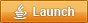Select File > Open. Drill down to the Model > CompositeStructureHierarchy > chameleon node. Select the entire Xholon model XML on this page (the large grey area). Drag it with your mouse so it’s over the chameleon node, and then release the mouse button. Click Refresh. Click Start, and a few seconds later click Pause. Display the line graph by clicking on View > xYChart.

The following figure shows some of the relevant features of the Xholon graphical user interface (GUI). The application is called Xholon Chameleon because initially it has only the Chameleon node (chameleon_0), a chameleon being an animal that can morph into something different. Each node includes an id such as 0 or 41. The File menu at the top includes an Open option. When you drag the Xholon XML from this Azimuth page to the Chameleon node, the entire ReactionNetworkSystem is inserted into the application, as shown here. It has exactly the same structure as the XML. The Start, Pause, and Refresh control nodes are along the top of the GUI. A line graph is displayed if you click on the Title:xYChart_90 node.

All nodes have a context menu that appears when you right-click the node. The figure shows the context menu for the AnalysisCRN node. It includes a Special node-specific submenu that can show various things associated with a reaction network (CRN), for example it can Show differential equations as described in this article. The context menu includes many other useful options, although not all are available with the online version of Xholon. Export, in the context menu for the ReactionNetworkSystem node, will display the system in a variety of text formats including SBML, SBGN, PNML, UML, and mind map (Freemind). It writes the text to the Java Console. The Special context submenu for the AnalysisPetriNet node includes an option to write out the Petri net in Python format.

The bottom line of the GUI displays summary information about the currently-selected node. The inputArc_85 node of the BE_AC transition has a reference to the B place node (labelled here as b_49). This input arc has a weight of 1.0.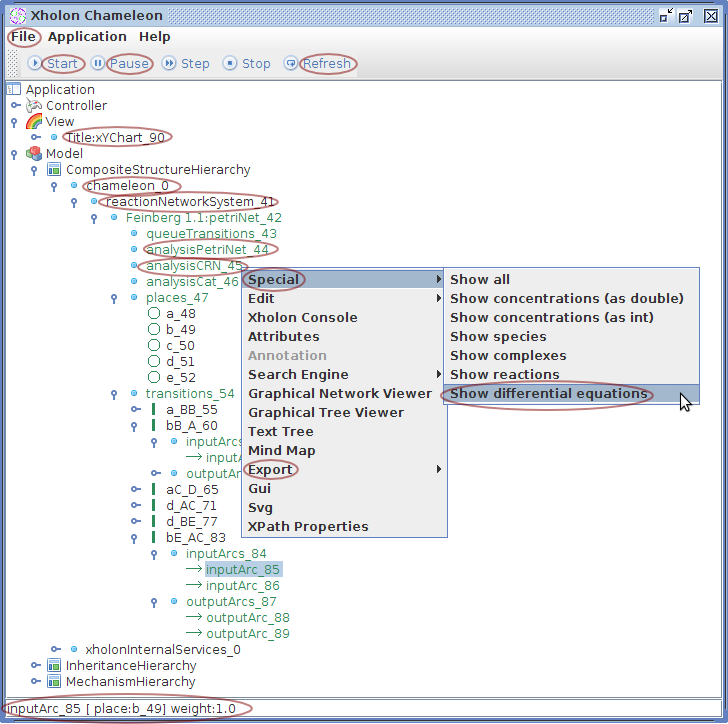To see the Java programming code behind any node in the Xholon application, right-click the node, and select Search Engine > Source Code . There are numerous other search engine options as well.

### Conclusion

This article has briefly explored Petri nets and reaction networks, which are essentially the same thing. Petri nets are useful on their own, but are also a well-defined relatively-simple starting point for understanding more complex approaches. We have seen how Petri nets can be automatically transformed into systems of differential equations, which, at least to me, are one of those more complex approaches. Future articles in this series will explore additional transformations beyond Petri nets.

category: blog